# Equation

(diff) ← Older revision | Latest revision (diff) | Newer revision → (diff)

An analytical form of the problem of investigating which values of variables in functions give equal results. The arguments on which the functions depend are usually called the unknowns, and the values of the unknowns for which the values of the function are equal are called the solutions of the equation; for such values of the unknowns one also says that they satisfy the given equation. A solutionof an equation of the form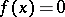is also called a root of.

The set of solutions of a given equation depends on the domainof values admitted for the unknown. An equation need not have a solution in; it is then said to be unsolvable in. If an equation is solvable, it may have one or several, or even an infinite set of, solutions. For example, the equation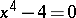is unsolvable in the domain of rational numbers, but it has two solutions: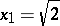,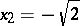in the domain of real numbers and four solutions,,,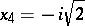in the domain of complex numbers. The equationhas infinitely many solutions: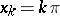,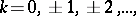in the domain of real numbers.

If an equation has as solution all numbers of a domain, then it is called an identity on.

A set of equations for which it is required to find values of the unknowns satisfying all equations simultaneously is called a system of equations; a set of values of the unknowns satisfying simultaneously all equations of the system is called a solution of the system. Two systems of equations (or two equations) are called equivalent if each solution of one system (equation) is a solution of the other system (equation), and conversely, where both systems (equations) are considered in one and the same domain.

The process of finding a solution of an equation usually consists in modifying it to an equivalent equation. In certain cases the given equation is reduced to another one for which the solution set is larger than that for the given equation. Therefore, if one performs this operation in solving an equation, it is possible for extra solutions to appear, and hence all solutions obtained from the transformed equation must be substituted in the original equation.

The most thoroughly studied are the algebraic equations (cf. Algebraic equation). Solving them was a principal task of algebra in the 16th century and 17th century. Ifis a transcendental function, then the equationis said to be transcendental; depending on the form of, it is called a trigonometric, logarithmic or exponential equation if these are the transcendental functions involved.

For the practical solution of equations one usually applies different approximate methods (cf., e.g., Linear algebra, numerical methods in).

Among systems of equations the simplest are systems of linear equations (cf. Linear equation). The solution of a system of equations (not necessarily linear) is reduced, generally speaking, to the solution of a single equation with the help of so-called elimination of the unknowns (cf. Elimination theory).

In number theory one considers equations over the integers, the study of the solutions of which forms the subject of the theory of Diophantine equations.

In the general case an equation is a list of problems the solution of which consists in finding elementsfrom a certain setsuch that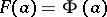, whereandare given mappings ofinto another set. Ifandare sets of numbers, there arise equations of the form considered above. Ifandare point sets in a higher-dimensional space, one obtains a system of equations. Ifandare sets of functions, then, depending on the character of the mapping, one obtains ordinary differential equations (cf. Differential equation, ordinary), partial differential equations (cf. Differential equation, partial), integral equations (cf. Integral equation), and other forms of equations.

How to Cite This Entry:
Equation. Encyclopedia of Mathematics. URL: http://encyclopediaofmath.org/index.php?title=Equation&oldid=18132
This article was adapted from an original article by Material from the article "Equation" in BSE-3 (originator), which appeared in Encyclopedia of Mathematics - ISBN 1402006098. See original article